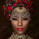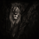3878 görüntülenme
Settings:
• FRAMA: blue line, SC = 252, FC = 40, length = 252
• EMA: orange line, length = 50

FRAMA seems to be the evolution of the current and much-used EMA . The basic strategy is simple: long if the price crosses up the line, short or exit if vice versa.
The main difference between EMA and FRAMA is that the first one seems to lag much more than the first one, as we can see from the chart below ( crude oil daily chart )

FYI
• http://etfhq.com/blog/2010/10/09/frama-is-it-effective/
```//@version=2

price = close
len = input(defval=252,minval=1)
FC = input(defval=40,minval=1)
SC = input(defval=252,minval=1)

w = log(2/(SC+1))
len1 = len/2
H1 = highest(high,len1)
L1 = lowest(low,len1)
N1 = (H1 - L1)/len1

H2 = for i = len1+1 to len
high>high[i]?high:high[i]

L2 = for i = len1+1 to len
low>low[i]?low:low[i]

N2 = (H2 - L2)/len1

H3 = highest(high,len)
L3 = lowest(low,len)
N3 = (H1 - L1)/len

dimen = (log(H1+H2) - log(H3))/log(2)
oldalpha = exp(w*(dimen-1))
oldN = (2-oldalpha)/oldalpha
newN = ((SC-FC)*(oldN-1)/(SC-1))+FC
alpha = 2/(newN+1)
out = nz(out)*(1-alpha) + price*alpha

plot(out,title="FRAMA")```

## YorumlarThis is quite clear on the cross in conjunction with the tenkan-sen and kijun-sen
Cevap GönderI was trying your script but it is somewhat different from my Sierra chart and Amibroker Frama. From what I know the final stage before the Frama calculation is the AMA, so I tried the Default settings of 16 and probably FC 2 and SC 30 but it is way different.
Here is the code from Ami which acts exactly the same with the code from Sierra. Could u give it a try

// FRAMA - Fractal Adaptive Moving Average
Price = (H+L)/2;
N = Param( "N", 16, 2, 40, 2 ); // must be even
N3 = ( HHV( High, N ) - LLV( Low, N ) ) / N;
HH = HHV( High, N / 2 ) ;
LL = LLV( Low, N / 2 );
N1 = ( HH - LL ) / ( N / 2 );
HH = HHV( Ref( High, - N/2 ), N/2 );
LL = LLV( Ref( Low, - N/2 ), N/ 2 );
N2 = ( HH - LL ) / ( N / 2 );
Dimen = IIf( N1 > 0 AND N2 > 0 AND N3 > 0, ( log( N1+N2) - log( N3 ) )/log( 2 ), Null );
alpha = exp( -4.6 * (Dimen -1 ) );
alpha = Min( Max( alpha, 0.01 ), 1 ); // bound to 0.01...1 range
Frama = AMA( Price, alpha );
Plot( Frama, "FRAMA("+N+")", ColorRGB(255,0,0), styleThick,maskAll );
_SECTION_END();
Cevap GönderJRA77
Thank you for your comment. My FRAMA contained some errors that I've corrected in latest version, but I dont know how to modify the published script.
Here the correct code, just copypaste on a new indicator if you want to use it (default settings are FC =1, SC =198, length =16, same as Ehlers's FRAMA):

//@version=2
price = input(hl2)
len = input(defval=16,minval=1)
FC = input(defval=1,minval=1)
SC = input(defval=198,minval=1)
len1 = len/2
w = log(2/(SC+1))
H1 = highest(high,len1)
L1 = lowest(low,len1)
N1 = (H1-L1)/len1
H2 = highest(high,len)
L2 = lowest(low,len)
N2 = (H2-L2)/len1
H3 = highest(high,len)
L3 = lowest(low,len)
N3 = (H3-L3)/len
dimen1 = (log(N1+N2)-log(N3))/log(2)
dimen = iff(N1>0 and N2>0 and N3>0,dimen1,nz(dimen1))
alpha1 = exp(w*(dimen-1))
oldalpha = alpha1>1?1:(alpha1<0.01?0.01:alpha1)
oldN = (2-oldalpha)/oldalpha
N = (((SC-FC)*(oldN-1))/(SC-1))+FC
alpha_ = 2/(N+1)
alpha = alpha_<2/(SC+1)?2/(SC+1):(alpha_>1?1:alpha_)
out = (1-alpha)*nz(out) + alpha*price
plot(out,title="FRAMA",color=blue,linewidth=2,transp=0)
Cevap GönderShizaru
Thank you very much for yur reply. Don't know you but really appreciate it.

line 26: Undeclared identifier `out`;
line 26: Cannot call `nz` with arguments (type_error); available overloads: nz(integer, integer) => integer; nz(float, float) => float; nz(float, series) => series; nz(series, float) => series; nz(series, series) => series; nz(float) => float; nz(series) => series;
line 26: Cannot call `operator *` with arguments (series, type_error); available overloads: *(integer, integer) => integer; *(float, float) => float; *(float, series) => series; *(series, float) => series; *(series, series) => series;
line 26: Cannot call `operator +` with arguments (type_error, series); available overloads: +(string, string) => string; +(integer, integer) => integer; +(float, float) => float; +(float, series) => series; +(series, float) => series; +(series, series) => series; +(integer) => integer; +(float) => float; +(series) => series;
line 27: Undeclared identifier `out`;
line 27: Cannot call `plot` with arguments (type_error, literal__string, color, literal__integer, literal__integer); available overloads: plot(series, string, series__color, integer, integer, bool, integer, float, integer, bool, series, literal__bool, string) => plot
Cevap GönderJRA77
pardon, there was an error in the out formula (nz(out) > nz(out) ).

Here the correct version:

//@version=2
price = input(hl2)
len = input(defval=16,minval=1)
FC = input(defval=1,minval=1)
SC = input(defval=198,minval=1)
len1 = len/2
w = log(2/(SC+1))
H1 = highest(high,len1)
L1 = lowest(low,len1)
N1 = (H1-L1)/len1
H2 = highest(high,len)
L2 = lowest(low,len)
N2 = (H2-L2)/len1
H3 = highest(high,len)
L3 = lowest(low,len)
N3 = (H3-L3)/len
dimen1 = (log(N1+N2)-log(N3))/log(2)
dimen = iff(N1>0 and N2>0 and N3>0,dimen1,nz(dimen1))
alpha1 = exp(w*(dimen-1))
oldalpha = alpha1>1?1:(alpha1<0.01?0.01:alpha1)
oldN = (2-oldalpha)/oldalpha
N = (((SC-FC)*(oldN-1))/(SC-1))+FC
alpha_ = 2/(N+1)
alpha = alpha_<2/(SC+1)?2/(SC+1):(alpha_>1?1:alpha_)
out = (1-alpha)*nz(out) + alpha*price
plot(out,title="FRAMA",color=blue,transp=0)
Cevap GönderShizaru
ok I found the problem :) when I write a number between bracket here, don't why but it disappears from the message.